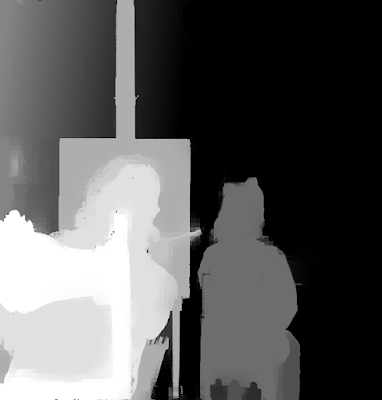## Friday, January 8, 2016

### 2D to 3D Conversion - David Bowie

To celebrate the birthday of David Bowie, here's a quick stereo conversion using Depth Map Automatic Generator 4 (DMAG4).Dense depth map obtained by DMAG4 using Max number of iterations = 5000 and Number of scales = 1.

That's a good example to show what increasing the number of scales can do. Here, we clearly have noise in the reference flat image.Dense depth map obtained by DMAG4 using Max number of iterations = 5000 and Number of scales = 3.

The dense depth map is smoother but we lost a bit of accuracy in the object boundaries. Doesn't really matter too much though.

## Wednesday, January 6, 2016

### 2D to 3D Conversion - Dali Painting Gala

The actual title of Dali's painting is "Dalí Seen from the Back Painting Gala from the Back Eternalized by Six Virtual Corneas Provisionally Reflected by Six Real Mirrors". Another 2d to 3d image conversion (stereo conversion) using Depth Map Automatic Generator 4 (DMAG4).

Of course, it took me a few iterations to arrive to this sparse depth map. The process is as follows: Start with as few brush strokes as possible, run DMAG4, run Wiggle Maker, identify the problems in the 3d wiggle, and go back to the sparse depth map. Iterate on this process until satisfied with the 3d wiggle. It usually takes 3 to 5 iterations.

As an alternative, one can also use Depth Map Automatic Generator 9 (DMAG9) to generate a dense depth map from the sparse depth map.Dense depth map produced by DMAG9 using 8 for the spatial sample rate and 32 for the color sample rate.

Here's a youtube video that summarizes the process of the stereo conversion:

## Tuesday, January 5, 2016

### 2D to 3D Conversion - Chevy Chase

It's time to do another 2d to 3d image conversion using Depth Map Automatic Generator 4 (DMAG4). To make it interesting, we are gonna look at the effect of increasing the number of scales. Recall that DMAG4 is based on Scale Space Random Walks (SSRW), which means that the number of scales used is at the heart of the method. In theory, a larger number of scales produces smoother depth maps but object boundaries might blur a little bit. When increasing the number of scales, it's pretty safe to reduce the number of iterations, if desired.Depth map obtained by DMAG4 using Number of iterations = 3000 and Number of scales = 1.Depth map obtained by DMAG4 using Number of iterations = 1000 and Number of scales = 3.

Here, I increased the number of scales by a factor of 3, so I reduced the number of iterations by the same factor (to save time).

It's interesting to see what Depth Map Automatic Generator 9 (DMAG9) can do on the exact same input (reference 2d image and sparse depth map).Depth map obtained by DMAG9 using Sample spatial rate = 4 and Sample color rate = 32.Depth map obtained by DMAG9 using Sample spatial rate = 8 and Sample color rate = 32.Depth map obtained by DMAG9 using Sample spatial rate = 16 and Sample color rate = 32.

The depth maps produced by DMAG9 are quite similar to the ones produced by DMAG4 but DMAG9 is much faster than DMAG4.

## Friday, January 1, 2016

### Quasi-Euclidean Uncalibrated Epipolar Rectification

Epipolar rectification transforms a pair of images so that the epipolar lines are parallel and horizontal and matching points have the same vertical coordinate. Euclidean epipolar rectification refers to the rectification of images produced by calibrated cameras, that is, images for which the camera matrix (intrinsic and extrinsic parameters) is known. Quasi-Euclidean epipolar rectification refers to the rectification of images taken by uncalibrated cameras with results comparable to the calibrated case.

Quasi-Euclidean Uncalibrated Epipolar Rectification by A. Fusiello and L. Irsara is a method that gives quite good results but the implementation details are a tad sketchy. Quasi-Euclidean Epipolar Rectification by Pascal Monasse goes into the details of implementation missing from the Fusiello-Irsara method. The following slides go a little bit further.

The input is a pair of images taken by uncalibrated cameras and a set of feature matches between the two images, typically obtained by Scale-invariant feature transform (SIFT) and Random sample consensus (RANSAC). The crux of the method is to find the two rotations to apply to the cameras (around their optical centers) as well as the focal length such that the Sampson error is minimized (by the Levenberg–Marquardt algorithm).

It might be a good idea to delve into inner workings of the Levenberg–Marquardt algorithm, a cross between the Gauss-Newton algorithm and Gradient descent: